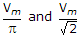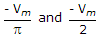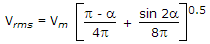# Electronics and Communication Engineering - Power Electronics - Discussion

### Discussion :: Power Electronics - Section 1 (Q.No.40)

40.

A single phase half wave rectifier is feeding a resistive load. Input voltage v = Vm sin ωt. The output dc voltage is Vdc and output rms voltage is Vrms . If firing angle is 180°, Vdc and Vrms respectively are

 [A]. 0 and 0 [B].[C]. 0 and - Vm [D].Explanation:

Put a = 180° in EqsOtherwise also a = 180° means no conduction.

Hence zero output voltage.